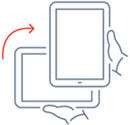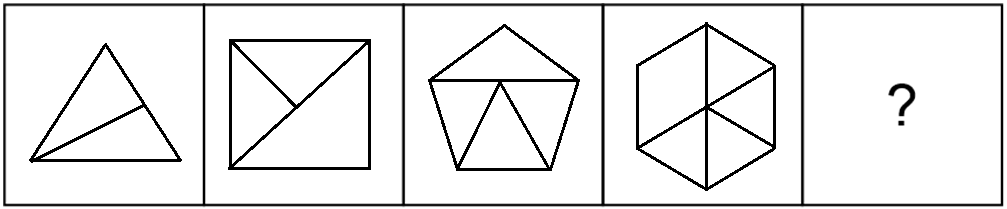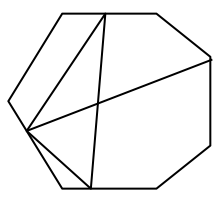We do not support landscape mode yet.
Please go back to portrait mode for the best experience
Question of The Day09-10-2019

Select the option which will come next in the given figure series?Correct Answer : b )Explanation :

Consider the given figure seriesIn the above figure series to obtain the next figure from the previous figure, we need to develop the following logic:

Logic 1: The next figure is obtained by increasing the number of sides of the previous figure by 1. As we can see the first figure is a triangle (number of sides ⇒3) and thus the next figure obtained is square (number of sides ⇒4)

Logic 2: The next figure is obtained by increasing the number of divisions in the previous figure by 1. As, we can see in the first figure number of divisions are 2 thus, in the next figure number of divisions are 3.

Considering option (b)Therefore, from the above logic, we can say that our missing figure will be containing 7 sides and 6 divisions.

Hence, (B) is the correct answer.

Such type of question is asked in various government exams like SSC CGL, SSC MTS, SSC CPO, SSC CHSL, RRB JE, RRB NTPC, RRB GROUP D, etc. Try and attempt free mock tests at PendulumEdu and boost your preparation to perform better on the D-day.